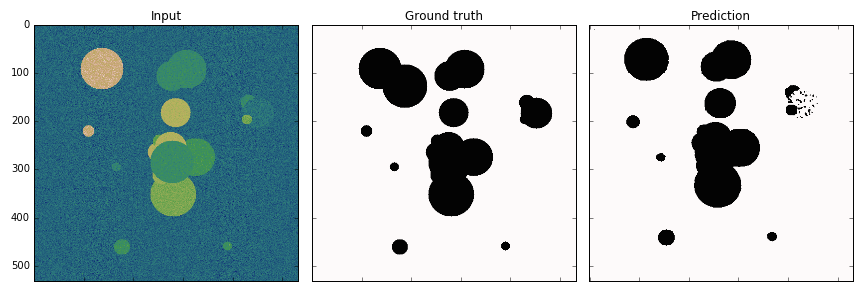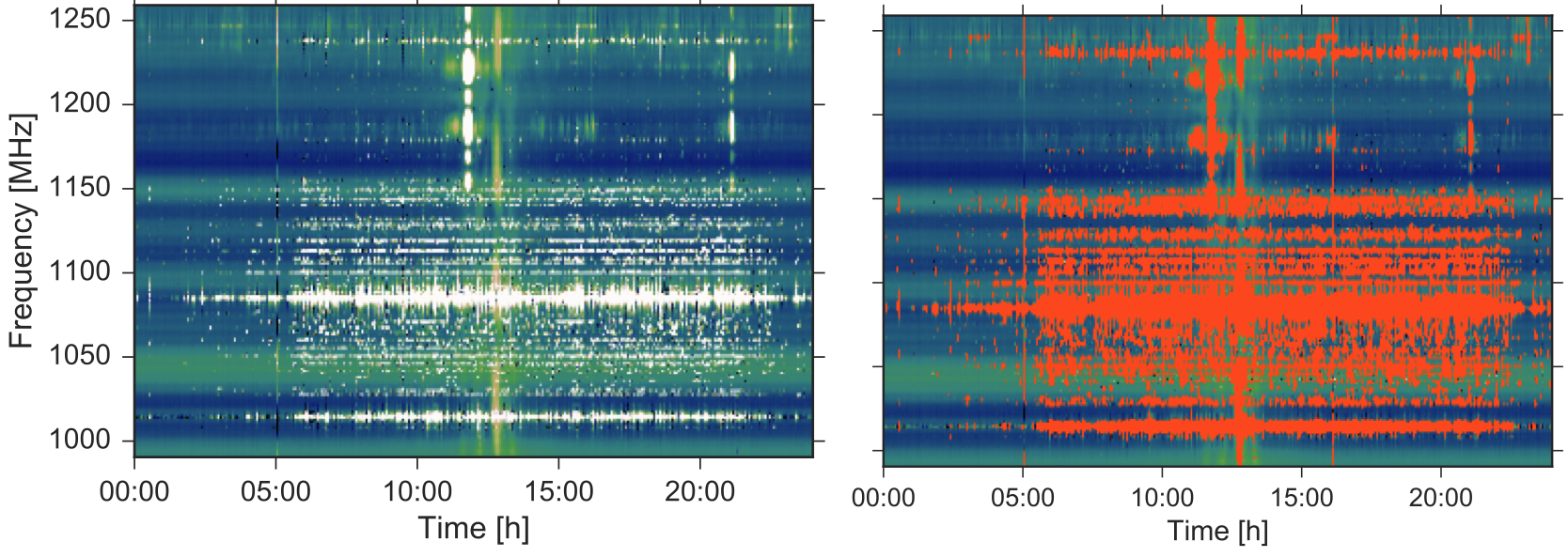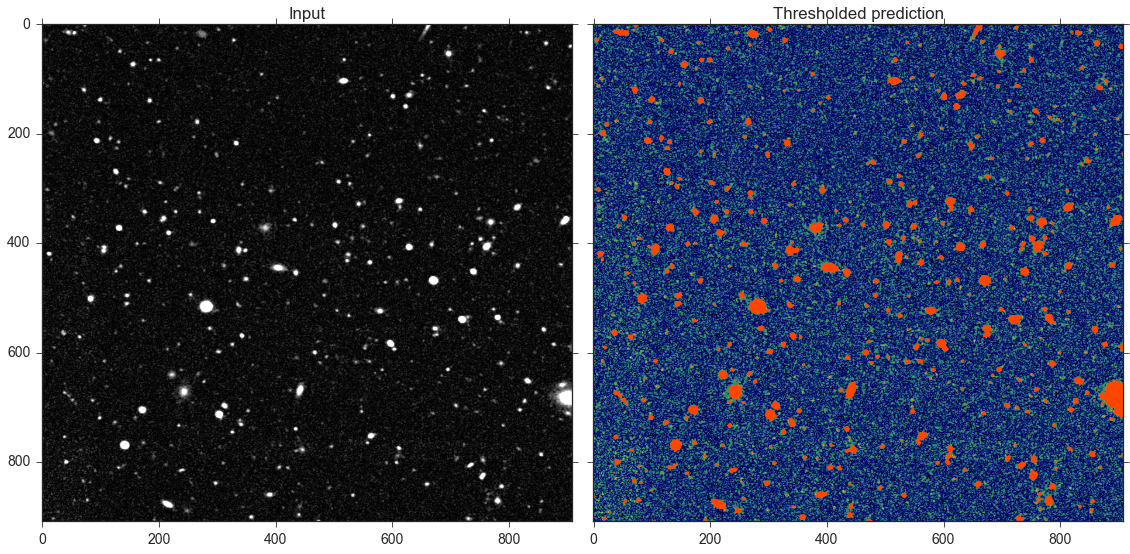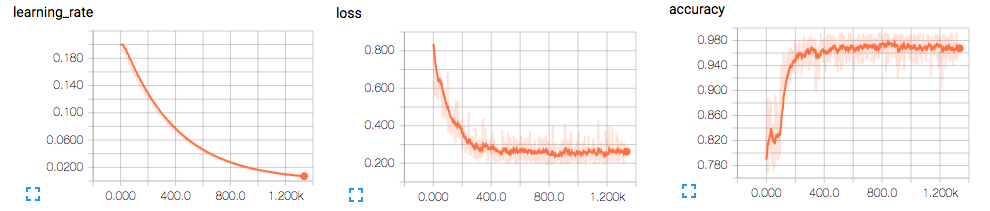# Tensorflow Unet¶This is a generic U-Net implementation as proposed by Ronneberger et al. developed with Tensorflow. The code has been developed and used for Radio Frequency Interference mitigation using deep convolutional neural networks .

The network can be trained to perform image segmentation on arbitrary imaging data. Checkout the Usage section or the included Jupyter notebooks for a toy problem or the Radio Frequency Interference mitigation discussed in our paper.

The code is not tied to a specific segmentation such that it can be used in a toy problem to detect circles in a noisy image.To more complex application such as the detection of radio frequency interference (RFI) in radio astronomy.Or to detect galaxies and star in wide field imaging data.As you use tf_unet for your exciting discoveries, please cite the paper that describes the package:

@article{akeret2017radio,
title={Radio frequency interference mitigation using deep convolutional neural networks},
author={Akeret, Joel and Chang, Chihway and Lucchi, Aurelien and Refregier, Alexandre},
journal={Astronomy and Computing},
volume={18},
pages={35--39},
year={2017},
publisher={Elsevier}
}


## Contents:¶

### Installation¶

The project is hosted on GitHub. Get a copy by running:

$git clone https://github.com/jakeret/tf_unet.git  Install the package like this: $ cd tf_unet
$pip install -r requirements.txt$ python setup.py install --user


Alternatively, if you want to develop new features:

$cd tf_unet$ python setup.py develop --user


Make sure TensorFlow is installed on your system. Installation instruction can be found here

### Usage¶

To use Tensorflow Unet in a project:

from tf_unet import unet, util, image_util

data_provider = image_util.ImageDataProvider("fishes/train/*.tif")

#setup & training
net = unet.Unet(layers=3, features_root=64, channels=1, n_class=2)
trainer = unet.Trainer(net)
path = trainer.train(data_provider, output_path, training_iters=32, epochs=100)

#verification
...

prediction = net.predict(path, data)

unet.error_rate(prediction, util.crop_to_shape(label, prediction.shape))

img = util.combine_img_prediction(data, label, prediction)
util.save_image(img, "prediction.jpg")


Keep track of the learning progress using Tensorboard. tf_unet automatically outputs relevant summaries.More examples can be found in the Jupyter notebooks for a toy problem or for a RFI problem. Further code is stored in the scripts folder.

### tf_unet Package¶

#### unet Module¶

Created on Jul 28, 2016

author: jakeret

class tf_unet.unet.Trainer(net, batch_size=1, verification_batch_size=4, norm_grads=False, optimizer=u'momentum', opt_kwargs={})[source]

Bases: object

Trains a unet instance

Parameters: net – the unet instance to train batch_size – size of training batch verification_batch_size – size of verification batch norm_grads – (optional) true if normalized gradients should be added to the summaries optimizer – (optional) name of the optimizer to use (momentum or adam) opt_kwargs – (optional) kwargs passed to the learning rate (momentum opt) and to the optimizer
output_epoch_stats(epoch, total_loss, training_iters, lr)[source]
output_minibatch_stats(sess, summary_writer, step, batch_x, batch_y)[source]
store_prediction(sess, batch_x, batch_y, name)[source]
train(data_provider, output_path, training_iters=10, epochs=100, dropout=0.75, display_step=1, restore=False, write_graph=False, prediction_path=u'prediction')[source]

Lauches the training process

Parameters: data_provider – callable returning training and verification data output_path – path where to store checkpoints training_iters – number of training mini batch iteration epochs – number of epochs dropout – dropout probability display_step – number of steps till outputting stats restore – Flag if previous model should be restored write_graph – Flag if the computation graph should be written as protobuf file to the output path prediction_path – path where to save predictions on each epoch
class tf_unet.unet.Unet(channels=3, n_class=2, cost=u'cross_entropy', cost_kwargs={}, **kwargs)[source]

Bases: object

A unet implementation

Parameters: channels – (optional) number of channels in the input image n_class – (optional) number of output labels cost – (optional) name of the cost function. Default is ‘cross_entropy’ cost_kwargs – (optional) kwargs passed to the cost function. See Unet._get_cost for more options
predict(model_path, x_test)[source]

Uses the model to create a prediction for the given data

Parameters: Returns prediction: model_path – path to the model checkpoint to restore x_test – Data to predict on. Shape [n, nx, ny, channels] The unet prediction Shape [n, px, py, labels] (px=nx-self.offset/2)
restore(sess, model_path)[source]

Restores a session from a checkpoint

Parameters: sess – current session instance model_path – path to file system checkpoint location
save(sess, model_path)[source]

Saves the current session to a checkpoint

Parameters: sess – current session model_path – path to file system location
tf_unet.unet.create_conv_net(x, keep_prob, channels, n_class, layers=3, features_root=16, filter_size=3, pool_size=2, summaries=True)[source]

Creates a new convolutional unet for the given parametrization.

Parameters: x – input tensor, shape [?,nx,ny,channels] keep_prob – dropout probability tensor channels – number of channels in the input image n_class – number of output labels layers – number of layers in the net features_root – number of features in the first layer filter_size – size of the convolution filter pool_size – size of the max pooling operation summaries – Flag if summaries should be created
tf_unet.unet.error_rate(predictions, labels)[source]

Return the error rate based on dense predictions and 1-hot labels.

tf_unet.unet.get_image_summary(img, idx=0)[source]

Make an image summary for 4d tensor image with index idx

#### image_util Module¶

author: jakeret

class tf_unet.image_util.BaseDataProvider(a_min=None, a_max=None)[source]

Bases: object

Abstract base class for DataProvider implementation. Subclasses have to overwrite the _next_data method that load the next data and label array. This implementation automatically clips the data with the given min/max and normalizes the values to (0,1]. To change this behavoir the _process_data method can be overwritten. To enable some post processing such as data augmentation the _post_process method can be overwritten.

Parameters: a_min – (optional) min value used for clipping a_max – (optional) max value used for clipping
channels = 1
n_class = 2
class tf_unet.image_util.ImageDataProvider(search_path, a_min=None, a_max=None, data_suffix=u'.tif', mask_suffix=u'_mask.tif', shuffle_data=True, n_class=2)[source]

Generic data provider for images, supports gray scale and colored images. Assumes that the data images and label images are stored in the same folder and that the labels have a different file suffix e.g. ‘train/fish_1.tif’ and ‘train/fish_1_mask.tif’

Usage: data_provider = ImageDataProvider(“..fishes/train/*.tif”)

Parameters: search_path – a glob search pattern to find all data and label images a_min – (optional) min value used for clipping a_max – (optional) max value used for clipping data_suffix – suffix pattern for the data images. Default ‘.tif’ mask_suffix – suffix pattern for the label images. Default ‘_mask.tif’ shuffle_data – if the order of the loaded file path should be randomized. Default ‘True’ channels – (optional) number of channels, default=1 n_class – (optional) number of classes, default=2
class tf_unet.image_util.SimpleDataProvider(data, label, a_min=None, a_max=None, channels=1, n_class=2)[source]

A simple data provider for numpy arrays. Assumes that the data and label are numpy array with the dimensions data [n, X, Y, channels], label [n, X, Y, classes]. Where n is the number of images, X, Y the size of the image.

Parameters: data – data numpy array. Shape=[n, X, Y, channels] label – label numpy array. Shape=[n, X, Y, classes] a_min – (optional) min value used for clipping a_max – (optional) max value used for clipping channels – (optional) number of channels, default=1 n_class – (optional) number of classes, default=2

#### util Module¶

Created on Aug 10, 2016

author: jakeret

tf_unet.util.combine_img_prediction(data, gt, pred)[source]

Combines the data, grouth thruth and the prediction into one rgb image

Parameters: data – the data tensor gt – the ground thruth tensor pred – the prediction tensor the concatenated rgb image
tf_unet.util.crop_to_shape(data, shape)[source]

Crops the array to the given image shape by removing the border (expects a tensor of shape [batches, nx, ny, channels].

Parameters: data – the array to crop shape – the target shape
tf_unet.util.plot_prediction(x_test, y_test, prediction, save=False)[source]
tf_unet.util.save_image(img, path)[source]

Writes the image to disk

Parameters: img – the rgb image to save path – the target path
tf_unet.util.to_rgb(img)[source]

Converts the given array into a RGB image. If the number of channels is not 3 the array is tiled such that it has 3 channels. Finally, the values are rescaled to [0,255)

Parameters: img – the array to convert [nx, ny, channels] the rgb image [nx, ny, 3]

#### layers Module¶

Created on Aug 19, 2016

author: jakeret

tf_unet.layers.bias_variable(shape, name=u'bias')[source]
tf_unet.layers.conv2d(x, W, b, keep_prob_)[source]
tf_unet.layers.crop_and_concat(x1, x2)[source]
tf_unet.layers.cross_entropy(y_, output_map)[source]
tf_unet.layers.deconv2d(x, W, stride)[source]
tf_unet.layers.max_pool(x, n)[source]
tf_unet.layers.pixel_wise_softmax(output_map)[source]
tf_unet.layers.weight_variable(shape, stddev=0.1, name=u'weight')[source]
tf_unet.layers.weight_variable_devonc(shape, stddev=0.1, name=u'weight_devonc')[source]

### Contributing¶

Contributions are welcome, and they are greatly appreciated! Every little bit helps, and credit will always be given.

You can contribute in many ways:

#### Types of Contributions¶

##### Report Bugs¶

If you are reporting a bug, please include:

• Your operating system name and version.
• Detailed steps to reproduce the bug.
##### Write Documentation¶

Tensorflow Unet could always use more documentation, whether as part of the official Tensorflow Unet docs, in docstrings, or even on the web in blog posts, articles, and such.

##### Submit Feedback¶

If you are proposing a feature:

• Explain in detail how it would work.
• Keep the scope as narrow as possible, to make it easier to implement.
• Remember that this is a volunteer-driven project, and that contributions are welcome :)

#### Pull Request Guidelines¶

Before you submit a pull request, check that it meets these guidelines:

1. The pull request should include tests.
2. If the pull request adds functionality, the docs should be updated. Put your new functionality into a function with a docstring, and add the feature to the list in README.rst.
3. The pull request should work for Python 2.6, 2.7, and 3.3, and for PyPy. make sure that the tests pass for all supported Python versions.

#### Tips¶

To run a subset of tests:

\$ py.test test/test_tf_unet.py